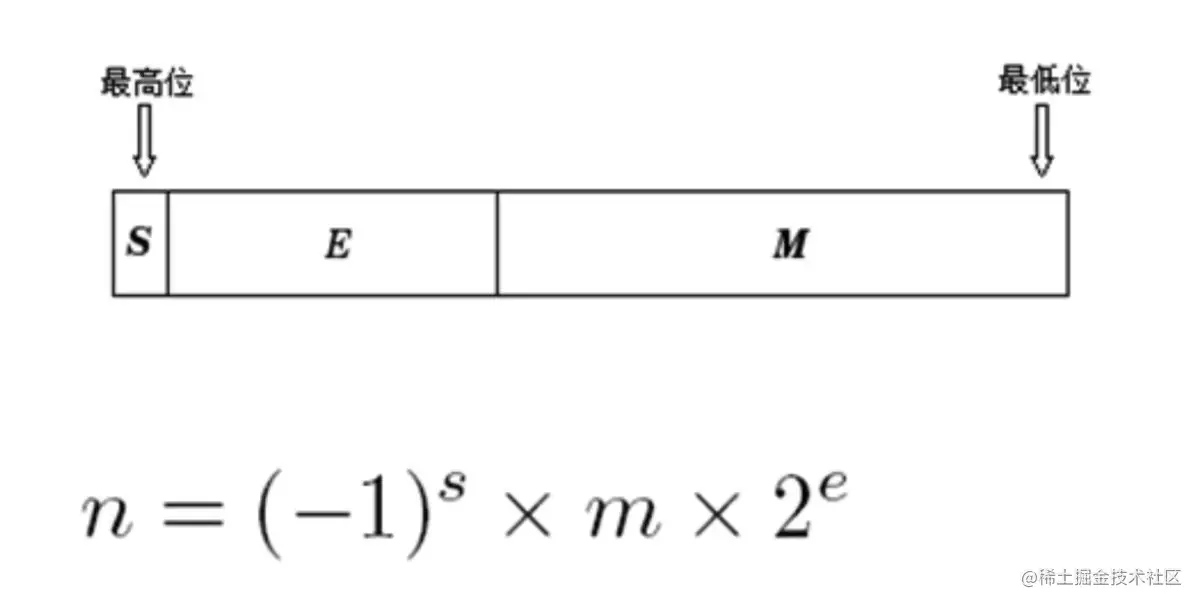# EasyC++07，C++中的浮点型

### 浮点数$1\le m < 2$

$(11.0)_2$

$1.1*2^1$

$s=1, m=1.1, e=1$

#### 关于m

$2^{-1} + 2^{-3}$

$(101)_2$

#### 关于e

1. e不全为0，或全为1时，采用上述的规则表示
2. e全为0时，e等于1-127，有效数字m不再默认加上1，这样是为了还原0.xxx的小数，以及接近于0的数
3. e全为1时，如果有效数字m全为0，表示无穷大，如果m不全为0，表示nan（not a number）

### 浮点数的使用

C++当中有两种浮点数的书写方式，第一种是使用常规的小数点表示法：

double a = 1.23;
float b = 3.43;

double a = 2.45e8;
double b = 1e-7;

2.45e8表示

$2.45 * 10^8$

### 注意事项

1. cout输出浮点数会删除结尾的0
2. 书写浮点数常量时默认为double类型，如果需要强制表示为float类型，请在结尾加上后缀f或者F，如：2.34f
3. 由于浮点数有精度，不能直接判断两个浮点数是否相等，很有可能得不到预期结果，正确的做法是判断精度范围，如：
double epsilon = 1e-8;
// 判断a是否和b相等
if (abs(a - b) < epsilon) {
// todo
}

1. 范围问题，如运行下列代码将得到错误的结果：
float a = 2.3e22f;
float b = a + 1.0f;

cout << b - a << endl;